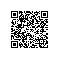# Scala入门到精通——第八节 包和引入

## 本节主要内容

1. 包的作用与定义
2. 包的作用域与引入（import）的使用方法
3. 访问控制
4. 包对象
5. import高级特性
6. 内部类

## 包的作用与定义

//将代码组织到cn.scala.xtwy包中
package cn.scala.xtwy

abstract class Animal {
//抽象字段(域）
var height:Int
//抽象方法
def eat:Unit
}

class Person(var height:Int) extends Animal{
override def eat()={
println("eat by mouth")
}

}

object Person extends App{
new Person(10).eat()
}

//下面的代码定义了一个cn.scala.xtwy包
//在程序的任何地方都可以通过cn.scala.xtwy.Teacher来使用Teacher这个类
package cn{
package scala{
package xtwy{
class Teacher {

}
}
}
}package cn{
package scala{
package xtwy{
class Teacher {

}
}
}
}
//添加了cn.scala.spark包，包中定义了一个SparkDemo类
package cn{
package scala{
package spark{
class SparkDemo{

}
}
}
}## 包的作用域与引入（import）的使用方法

package cn{
package scala{
//在包cn.scala下创建了一个Utils单例
object Utils{
def toString(x:String){
println(x)
}
//外层包无法直接访问内层包，下面这一行代码编译通不过
//def getTeacher():Teacher=new Teacher("john")
//如果一定要使用的话，可以引入包
import cn.scala.xtwy._
def getTeacher():Teacher=new Teacher("john")
}
//定义了cn.scala.xtwy
package xtwy{
class Teacher(var name:String) {
//演示包的访问规则
//内层包可以访问外层包中定义的类或对象，无需引入
def printName()={Utils.toString(name)}
}

}
}
}
object appDemo{
//scala允许在任何地方进行包的引入，_的意思是引入该包下的所有类和对象
import cn.scala._
import cn.scala.xtwy._
def main(args: Array[String]): Unit = {
Utils.toString(new Teacher("john").name)
new Teacher("john").printName()
}

}

## 访问控制

1 private 成员
private成员同java是一样的，所有带该关键字修饰的成员仅能在定义它的类或对象中使用，在外部是不可见的

class Student(var name:String,var age:Int){
private var sex:Int=0
//内部类
class Course(val cName:String,val gpa:Float){
//可以直接访问其外部类的私有成员
def getStudentSex(student:Student)= student.sex
}
}

//班级类
class Class{
//下面这条语句统计通不过，因为sex是私有的
// def getStudentSex(student:Student)=student.sex
}
object Student {
private var studentNo:Int=0;
def uniqueStudentNo()={
studentNo+=1
studentNo
}
def apply(name:String,age:Int)=new Student(name,age)

def main(args: Array[String]): Unit = {
println(Student.uniqueStudentNo())
val s=new Student("john",29)
//直接访问伴生类Student中的私有成员
println(s.sex)

val s1=Student("john",29)
println(s1.name)
println(s1.age)

//使用内部类
val c1=new s1.Course("Scala",3.0f)

}
}


2 protected 成员

class SuperClass {
protected def f()=println(".....")
}

class SubClass extends SuperClass{
f()
}

class OtherClass{
//下面这个语句会报错
//f()
}

3 无修饰符成员

4 范围保护

package cn{
class UtilsTest{
//编译通不过，因为Utils利用private[scala]修饰，只能在scala及其子包中使用
//Utils.toString()
}
package scala{
//private[scala]限定Utils只能在scala及子包中使用
private[scala] object Utils{
def toString(x:String){
println(x)
}
import cn.scala.xtwy._
def getTeacher():Teacher=new Teacher("john")

}
package xtwy{
class Teacher(var name:String) {
def printName()={Utils.toString(name)}
}

}
}
}
object appDemo{
import cn.scala._
import cn.scala.xtwy._
def main(args: Array[String]): Unit = {
//编译通不过，同UtilsTest
//Utils.toString(new Teacher("john").name)
new Teacher("john").printName()
}

}

private[this]，限定只有该类的对象才能访问，称这种成员为对象私有成员

package cn.scala.xtwy;

class Teacher(var name: String) {
private[this] def printName(tName:String="") :Unit= { println(tName) }
//调用private[this] printName方法
def print(n:String)=this.printName(n)
}

object Teacher{
//private[this]限定的成员，即使伴生对象Teacher也不能使用
//def printName=new Teacher("john").printName()
}

object appDemo {
def main(args: Array[String]): Unit = {
//编译不能通过
//new Teacher("john").printName()
}

}

private，定义的类及伴生对象可以访问

package cn.scala.xtwy;

class Teacher(var name: String) {
private def printName(tName:String="") :Unit= { println(tName) }
//可以访问
def print(n:String)=this.printName(n)
}

object Teacher{
//伴生对象可以访问
def printName=new Teacher("john").printName()
}

object appDemo {
def main(args: Array[String]): Unit = {
//不能访问
//new Teacher("john").printName()
}

}


private[scala] 在定义的类中可以访问，在scala包及子包中可以访问
private[this] 只能在定义的类中访问，即使伴生对象也不能访问团
private 在定义的的类及伴生对象中可以访问，其它地方不能访问
protected[scala] 在定义的类及子类中可以访问，在scala包及子包中可以访问，
protected[this] 只能在定义的类及子类中访问，即使伴生对象也不能访问
protected 在定义的类及子类中访问，伴生对象可以访问，其它地方不能访问

## 包对象

//下面的代码给出了包对象的定义
package cn.scala.xtwy

//利用package关键字定义单例对象
package object Math {
val PI=3.141529
val THETA=2.0
val SIGMA=1.9
}

class Coputation{
def computeArea(r:Double)=Math.PI*r*r
}D:\ScalaWorkspace\ScalaChapter08\bin\cn\scala\xtwy\Math>javap -private package.c
lass
Compiled from "Math.scala"
public final class cn.scala.xtwy.Math.package {
public static double SIGMA();
public static double THETA();
public static double PI();
}

D:\ScalaWorkspace\ScalaChapter08\bin\cn\scala\xtwy\Math>javap -private package$. class Compiled from "Math.scala" public final class cn.scala.xtwy.Math.package$ {
public static final cn.scala.xtwy.Math.package$MODULE$;
private final double PI;
private final double THETA;
private final double SIGMA;
public static {};
public double PI();
public double THETA();
public double SIGMA();
private cn.scala.xtwy.Math.package\$();
}

## import高级特性

1 隐式引入

scala._
Predef._

2 重命名

scala中允许对引入的类或方法进行重命名，如果我们需要在程序中同时使用java.util.HashMap及scala.collection.mutable.HashMap时，可以利用重命名的方法消除命名冲突的问题，虽然也可以采用包名前缀的方式使用，但代码不够简洁

//将java.util.HashMap重命名为JavaHashMap
import java.util.{ HashMap => JavaHashMap }
import scala.collection.mutable.HashMap
object RenameUsage {
def main(args: Array[String]): Unit = {
val javaHashMap = new JavaHashMap[String, String]()
javaHashMap.put("Spark", "excellent")
javaHashMap.put("MapReduce", "good")
for(key <- javaHashMap.keySet().toArray){
println(key+":"+javaHashMap.get(key))
}

val scalaHashMap=new HashMap[String,String]
scalaHashMap.put("Spark", "excellent")
scalaHashMap.put("MapReduce", "good")
scalaHashMap.foreach(e=>{
val (k,v)=e
println(k+":"+v)
})
}

}

3 类隐藏

//通过HashMap=> _，这样类便被隐藏起来了
import java.util.{HashMap=> _,_}
import scala.collection.mutable.HashMap

object RenameUsage {
def main(args: Array[String]): Unit = {

//这样的话,HashMap便无歧义地指向scala.collection.mutable.HashMap
val scalaHashMap=new HashMap[String,String]
scalaHashMap.put("Spark", "excellent")
scalaHashMap.put("MapReduce", "good")
scalaHashMap.foreach(e=>{
val (k,v)=e
println(k+":"+v)
})
}


## 内部类

class OuterClass {
//即使定义为 private[this] var x:Int=0，也是可行的
private var x:Int=0

//def getInnerY=y，外部类不能直接访问内部类的成员域
class InnerClass{
private var y:Int=0
//内部的类可以直接访问外部类的成员变量和成员方法,注意外部类的成员变量是private
def getOuterX= x
}
}

object AppDemo{
def main(args: Array[String]): Unit = {
val o=new OuterClass
//创建内部类对象，通过o.InnerClass方式，InnerClass就像是OuterClass的成员变量一样
val i=new o.InnerClass
println(i.getOuterX)
}
}使用钉钉扫一扫加入圈子
+ 订阅